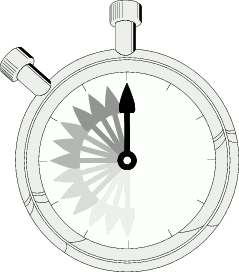# Estimating Timing

We want to estimate the required acceleration time period.

## TimingThis crude approximation will confirm if the LC timing estimates are in the appropriate range.

Assume:

• Projectile moves 2 inches under const acceleration, to speed v = 50 fps or to v = 200 fps.
• Find the time t

Solution:

1. Distance d = at2/2
Solving this for t yields: t = sqrt(2d/a)
2. Also, from classical physics, we know v = at
Solving this for a yields: a = v/t

Put 1) and 2) together to get: t = 2d/v

At 50 fps:

• t = (2)(0.0508 m)/(15.24 m/s) = 6.67 ms

At 200 fps:

• t = (2)(0.0508 m)/(60.96 m/s) = 1.67 ms

This means that a current pulse between 1 and 7 milliseconds is necessary to match the required acceleration time.

This timing does fit well into the energy calculations used elsewhere.

 < Previous Page 7 of 37 Next >